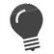# %DECML

Convert an ASCII character to its decimal value

 WTSupported in traditional Synergy on Windows WNSupported in Synergy .NET on Windows USupported on UNIX VSupported on OpenVMS
```result = %DECML(expression)
```

or

```xcall DECML(expression, result)
```

Return value

result

The decimal equivalent of the converted character. If expression is null, result will be zero. (n)

Arguments

expression

The alpha expression whose leftmost character is to be converted to a decimal ASCII value. (a)

Discussion

The DECML subroutine converts a single ASCII character into its corresponding decimal value and returns or stores the value in the specified result variable. (See Appendix B: ASCII Character Set for a complete set of ASCII characters and their decimal equivalents.)

The maximum decimal value is 255; the size of result must be large enough or truncation will occur.If sign is not important (characters greater than 127 are not used), it’s more efficient to cast a single-character variable as an integer or overlay the first character with an i1 to access its ASCII value. For example:```record achar ,a1 asc_value ,i1 @achar ```

Examples

```record
achar       ,a3
dchar       ,d3
proc
xcall decml("A1\$", dchar)                           ;dchar = 065 ("A")
achar = "t-R"
xcall decml(achar, dchar)                           ;dchar = 116 ("t")
xcall decml(achar(2,2), dchar)                      ;dchar = 045 ("-")
stop
end
```

The following subroutine builds a script filename from a partial filename and uppercases it if it is lowercase.

```subroutine bldnam
a_name      ,a
record
ix          ,d3
proc
if (.not.(%instr(2, a_name, ".")))                  ;If no extension, add one
begin
a_name((%trim(a_name) + 1):4) = ".wsc"
if (%instr(1, a_name, ":", ix)) then            ;If filename is uppercase,
ix = %decml(a_name(ix+1:1))                   ; uppercase extension too.
else
ix = %decml(a_name)
case ix of
begincase
65 - 90: upcase a_name
endcase
clear ix
end
return
endsubroutine```